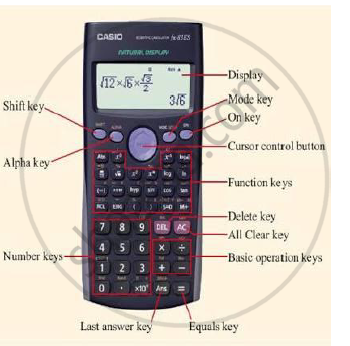# Describe Any One of the Following Objects Giving Definition Diagram Component and Working of Calculator Or Voltmeter. ( - Communication Skills

Definition

Describe the following objects giving definition diagram component and working of calculator.

#### Solution

Calculator
Definition: A calculator is a small portable, electronic device used to perform both basic and complex operations of arithmetic.Components: In general, a basic electronic calculator consists of the following component:

1.  Power source (battery or solar cell).
2.  Keypad (keys used to input number).
3.  Function commands are basic operation keys (addition multiplication) etc.
4.  Processor chip
5.  Encoder unit (converts number and functions into binary code).
6.  X register and Y register (temporary stores while calculating).
7. Decoder unit (convert binary code into "decimal" number which can be displayed on the display unit).
8.  Display panel (Display input number, commands and results calculates usually have liquid crystal display).
Working: calculator work by processing information in binary form. When we input a number into a calculator, an integrated circuit converts those number to binary string of zeros and ones. The ICS then use the strings to turn transistor on and off with electricity to perform the Desire calculations. 100 calculation has been completed, the answer in binary form is been converted back to the normal base 10 system and display on the calculator display screen.
Concept: Information Communication Technology (Ict) Enabled Communication Media
Is there an error in this question or solution?
2017-2018 (December) CBCGS

Share